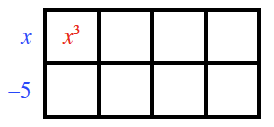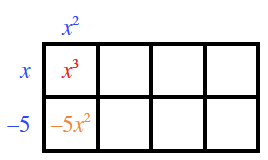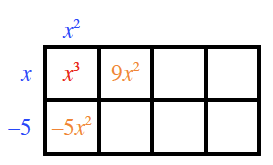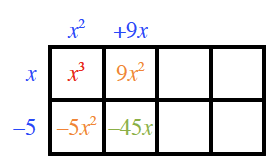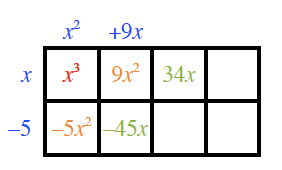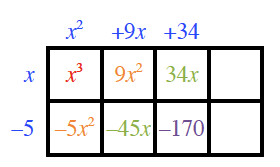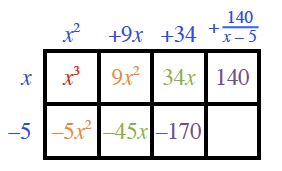### Home > PC3 > Chapter 4 > Lesson 4.1.2 > Problem4-29

4-29.

Consider the polynomial $p(x)=x^3+4x^2-11x-30$.

1. If you want to determine the factors of $p(x)$, is $x-5$ a reasonable guess for one of the factors? Explain.

Is $5$ a factor of the last term ($30$)?

2. How can you determine if $x-5$ is a factor? Can you think of more than one way?

3. Is $x-5$ a factor of $x^3+4x^2-11x-30$?

Set up the area model with the divisor on the left of the box. Input only the first term of the dividend.

Generic rectangle, 2 rows, 4 columns, left edge labeled, x minus 5, interior top left, labeled x cubed.

Since $x$ divides into $x^3$, $x^2$ times, write the $x^2$ on top and multiply $x^2$ with the $−5$ and fill in the area model with the product.

Labels added, top edge left, x squared, interior bottom left, negative 5, x squared.

Add an expression to $−5x^2$ in order to get the needed $4x^2$. Add it to the right of $x^3$.

Label added, interior top, second from left, 9 x squared.

Dividing $x$ into $9x^2$ gives $9x$. Add the $9x$ at the top. Then multiply $9x$ and $−5$ and fill the answer in the area model.

Labels added: top edge, second from left, + 9 x, interior bottom, second from left, negative 45 x.

Add an expression to $−45x$ in order to get the needed $−11x$. Add it to the right of $9x^2$.

Label added: interior top, second from right, 34 x.

Dividing $x$ into $34x$ gives $34$. Add the $34$ at the top. Then multiply $34$ and $−5$ and fill the answer in the area model.

Labels added: top edge, second from right, + 34, interior bottom, second from right, negative 170.

Add an expression to $−170$ in order to get the needed $−30$. Add it to the right of $34x$. This is the remainder.

Labels added: top edge, right, 140 divided by quantity x minus 5. Interior top right, 140.